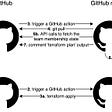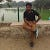# Boolean

• Logical : `!` (logical negation), `&&` (AND), `||` (OR)
• Comparisons : `==` (equality), `!=` (inequality)

# Integer

• Comparisons: `<=`, `<`, `==`, `!=`, `>=` and `>`
• Bit operators: `&`, `|`, `^` (bitwise exclusive or) and `~` (bitwise negation)
• Arithmetic operators: `+`, `-`, unary `-`, unary `+`, `*`, `/`, `%`, `**` (exponentiation), `<<` (left shift) and `>>` (right shift)

• Comparisons: `<=`, `<`, `==`, `!=`, `>=` and `>`

## balance

• `<address>.balance (uint256)`: balance of the Address in Wei

## transfer and send

• `<address>.transfer(uint256 amount)`: send given amount of Wei to Address, throws on failure
• `<address>.send(uint256 amount) returns (bool)`: send given amount of Wei to Address, returns false on failure

## call

• `<address>.call(...) returns (bool)`: issue low-level CALL, returns false on failure

## delegatecall

• `<address>.delegatecall(...) returns (bool)`: issue low-level DELEGATECALL, returns false on failure
`contract A {  uint value;  address public sender;  address a = "0x"; // address of contract B  function makeDelegateCall(uint _value) {    a.delegatecall(bytes4(keccak256("setValue(uint)")), _value); // Value of A is modified  }}contract B {  uint value;  address public sender;  setValue(uint _value) {    value = _value;    sender = msg.sender; // msg.sender is preserved in delegatecall. It was not available in callcode.  }}`

## callcode

• `<address>.callcode(...) returns (bool)`: issue low-level CALLCODE, returns false on failure

# Array

`uint[] dynamicSizeArray;uint fixedSizeArray;`

# Fixed byte arrays

• `.length` : read-only

# Enum

`enum ActionChoices {   GoLeft,   GoRight,   GoStraight,   SitStill }ActionChoices choice = ActionChoices.GoStraight;`

# Struct

`struct Funder {    address addr;    uint amount;}Funder funders;`

# Control Structures

• `if` `else`
• `while`
• `do`
• `for`
• `break`
• `continue`
• `return`
• `? :`

# Access modifiers

• `public` - Accessible from this contract, inherited contracts and externally
• `private` - Accessible only from this contract
• `internal` - Accessible only from this contract and contracts inheriting from it
• `external` - Cannot be accessed internally, only externally. Recommended to reduce gas. Access internally with `this.f`.

# Parameters

## Input parameters

`function f(uint _a, uint _b) {}`

## Output parameters

`function f(uint _a, uint _b) returns (uint _sum) {   _sum = _a + _b;}`

# Constructor

`contract C {   address owner;   uint status;   constructor(uint _status) {       owner = msg.sender;       status = _status;   }}`

# Function Calls

## Internal Function Calls

`contract C {    function funA() returns (uint) {        return 5;     }        function FunB(uint _a) returns (uint ret) {        return funA() + _a;     }}`

## Named Calls

`function f(uint a, uint b) {  }function g() {    f({b: 1, a: 2});}`

## Unnamed function parameters

`function f(uint a, uint) returns (uint) {    return a;}`

# Function type

`pragma solidity ^0.4.18;contract Oracle {  struct Request {    bytes data;    function(bytes memory) external callback;  }  Request[] requests;  event NewRequest(uint);  function query(bytes data, function(bytes memory) external callback) {    requests.push(Request(data, callback));    NewRequest(requests.length - 1);  }  function reply(uint requestID, bytes response) {    // Here goes the check that the reply comes from a trusted source    requests[requestID].callback(response);  }}contract OracleUser {  Oracle constant oracle = Oracle(0x1234567); // known contract  function buySomething() {    oracle.query("USD", this.oracleResponse);  }  function oracleResponse(bytes response) {    require(msg.sender == address(oracle));  }}`

# Function Modifier

`modifier onlyOwner {    require(msg.sender == owner);    _;}function close() onlyOwner {    selfdestruct(owner);}`

# View or Constant Functions

`function f(uint a) view returns (uint) {    return a * b; // where b is a storage variable}`

# Pure Functions

`function f(uint a) pure returns (uint) {    return a * 42;}`

# Fallback Function

`function() {  // Do something}`

# Creating contracts using `new`

`contract A {    function add(uint _a, uint _b) returns (uint) {        return _a + _b;    }}contract C {    address a;    function f(uint _a) {        a = new A();    }}`

# Contract Inheritance

`contract owned {    function owned() { owner = msg.sender; }    address owner;}contract mortal is owned {    function kill() {        if (msg.sender == owner) selfdestruct(owner);    }}contract final is mortal {    function kill() {         super.kill(); // Calls kill() of mortal.    }}`

## Multiple inheritance

`contract A {}contract B {}contract C is A, B {}`

## Constructor of base class

`contract A {    uint a;    constructor(uint _a) { a = _a; }}contract B is A(1) {    constructor(uint _b) A(_b) {    }}`

# Abstract Contracts

`pragma solidity ^0.4.0;contract A {    function C() returns (bytes32);}contract B is A {    function C() returns (bytes32) { return "c"; }}`

# Interface

• Cannot have any functions implemented.
• Cannot inherit other contracts or interfaces.
• Cannot define constructor.
• Cannot define variables.
• Cannot define structs.
• Cannot define enums.
`pragma solidity ^0.4.11;interface Token {    function transfer(address recipient, uint amount);}`

# Events

`pragma solidity ^0.4.0;contract ClientReceipt {    event Deposit(        address indexed _from,        bytes32 indexed _id,        uint _value    );    function deposit(bytes32 _id) payable {        emit Deposit(msg.sender, _id, msg.value);    }}`

# Library

`library arithmatic {    function add(uint _a, uint _b) returns (uint) {        return _a + _b;    }}contract C {    uint sum;    function f() {        sum = arithmatic.add(2, 3);    }}`

# Using — For

`library arithmatic {    function add(uint _a, uint _b) returns (uint) {        return _a + _b;    }}contract C {    using arithmatic for uint;        uint sum;    function f(uint _a) {        sum = _a.add(3);    }}`

# Error Handling

• `assert(bool condition)`: throws if the condition is not met - to be used for internal errors.
• `require(bool condition)`: throws if the condition is not met - to be used for errors in inputs or external components.
• `revert()`: abort execution and revert state changes
`function sendHalf(address addr) payable returns (uint balance) {    require(msg.value % 2 == 0); // Only allow even numbers    uint balanceBeforeTransfer = this.balance;    addr.transfer(msg.value / 2);    assert(this.balance == balanceBeforeTransfer - msg.value / 2);    return this.balance;}`

# Block variables

• `block.blockhash(uint blockNumber) returns (bytes32)`: hash of the given block - only works for the 256 most recent blocks excluding current
• `block.coinbase (address)`: current block miner’s address
• `block.difficulty (uint)`: current block difficulty
• `block.gaslimit (uint)`: current block gaslimit
• `block.number (uint)`: current block number
• `block.timestamp (uint)`: current block timestamp as seconds since unix epoch
• `now (uint)`: current block timestamp (alias for `block.timestamp`)

# Transaction variables

• `msg.data (bytes)`: complete calldata
• `msg.gas (uint)`: remaining gas
• `msg.sender (address)`: sender of the message (current call)
• `msg.sig (bytes4)`: first four bytes of the calldata (i.e. function identifier)
• `msg.value (uint)`: number of wei sent with the message
• `tx.gasprice (uint)`: gas price of the transaction
• `tx.origin (address)`: sender of the transaction (full call chain)

# Mathematical and Cryptographic Functions

• `addmod(uint x, uint y, uint k) returns (uint)`: compute (x + y) % k where the addition is performed with arbitrary precision and does not wrap around at 2**256.
• `mulmod(uint x, uint y, uint k) returns (uint)`: compute (x * y) % k where the multiplication is performed with arbitrary precision and does not wrap around at 2**256.
• `keccak256(...) returns (bytes32)`: compute the Ethereum-SHA-3 (Keccak-256) hash of the (tightly packed) arguments
• `sha256(...) returns (bytes32)`: compute the SHA-256 hash of the (tightly packed) arguments
• `sha3(...) returns (bytes32)`: alias to keccak256
• `ripemd160(...) returns (bytes20)`: compute RIPEMD-160 hash of the (tightly packed) arguments
• `ecrecover(bytes32 hash, uint8 v, bytes32 r, bytes32 s) returns (address)`: recover the address associated with the public key from elliptic curve signature or return zero on error (example usage)

# Contract Related

• `this (current contract’s type)`: the current contract, explicitly convertible to Address
• `selfdestruct(address recipient)`: destroy the current contract, sending its funds to the given Address
• `suicide(address recipient)`: alias to selfdestruct. Soon to be deprecated.

--

--

--

## More from ABHISHEK KUMAR

Love podcasts or audiobooks? Learn on the go with our new app.

## Handy Log File Management Tools to View and Monitor Your Logs## Using Terraform on GitHub Actions to Manage Team Membership on GitHub## Object Oriented Concepts (OOC)## Why We Migrated from Exadata to a Scale-Out HTAP Database for Near Real-Time Analytics## 5 top android libraries to explore in April 2020## 鬼滅之刃劇場版 無限列車篇線上看 | 最新電影 2020| 小鴨影音| HD 4K | BLURAY## dApps 101: How to start dApps Development## How to Optimize Solidity Smart Contracts Effectively!## Types of Smart Contract Tests #1 Introduction## Applications of Blockchain: Delving into Parametric Insurance.